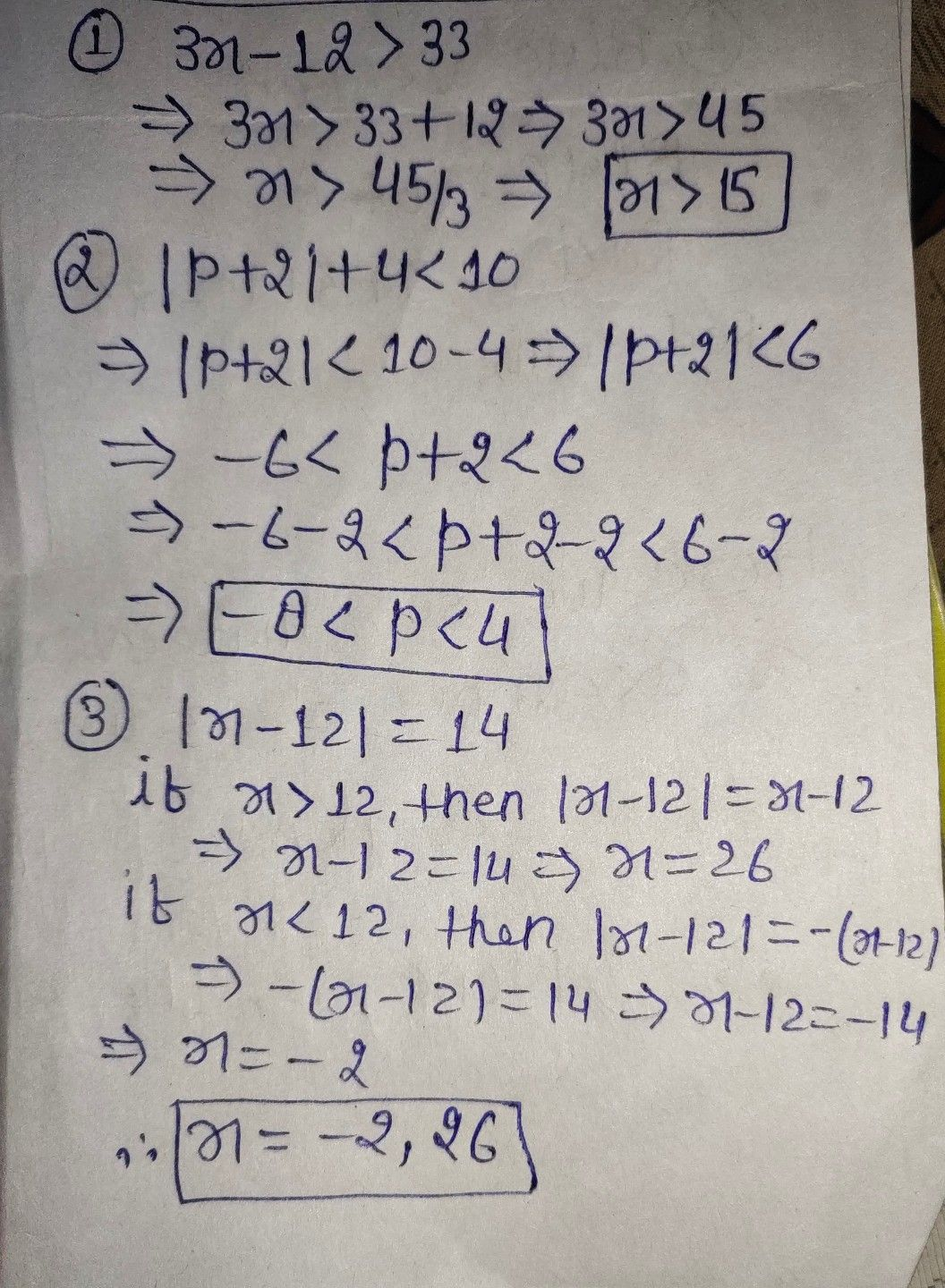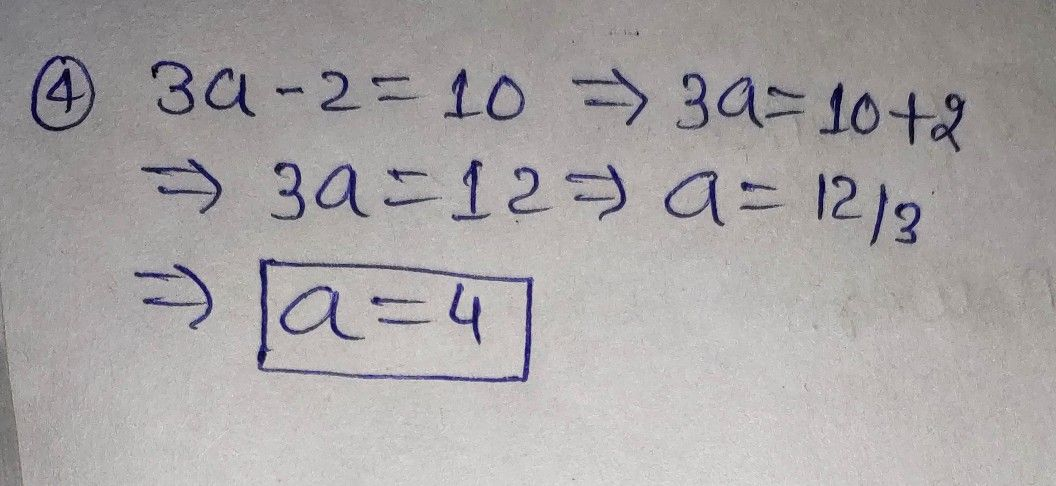Symbol
Problem$3x-12>33^{+}$ 1 point $○\left(x>-15\right)$ $○\left(x>15\right)$ $○\left(x<-15\right)$ $○\left(x<15\right)$ Solve: $|p+2|+4<10≠$ 1 point $-8<p<4$ $8<p<-4$ $-8<p<-4$ $8<p<4$ Which of the following is equivalent $to|x-12|=147≠$ 1 point $\left(2,26\right)$ $\left(2,-26\right)$ $\left(-2,26\right)$ $\left(-2,-26\right)$ $3a-2=10≠$ 1 point $a=2$ $a=4$ a = 6 O a = 8 O O This is a required question